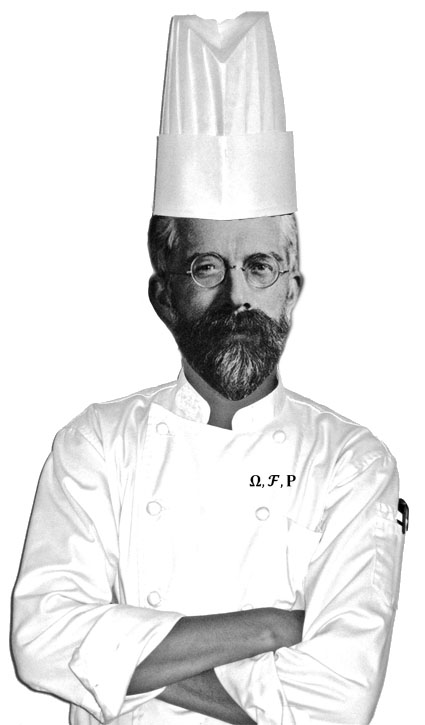31
Jul 13

A probability cookbookRandomness – Probability = Chance

Chance – Randomness = Fate

Fate + God = Predestination

Probability + Epistemology = Types of Randomness

Subjective Probability = Betting + Coherence

Propensity theory = Probability + Animism

Kolmogorov Axioms = Probability – randomness – chance

Probability + Complexity = Cryptography

Chaos + Ignorance = Randomness

Regression: Data = Signal + Noise

Bayesian:
Posterior = Prior $\times$ Likelihood
Prior + Data $\rightarrow$ Probability

Probabilitst:
Probability $\rightarrow$ Frequency

Statistical:
Frequency $\rightarrow$ Probability

Big Data:
Predictive value $\gg$ Model simplicity
High dimensions + Fast computers = De chao ordo